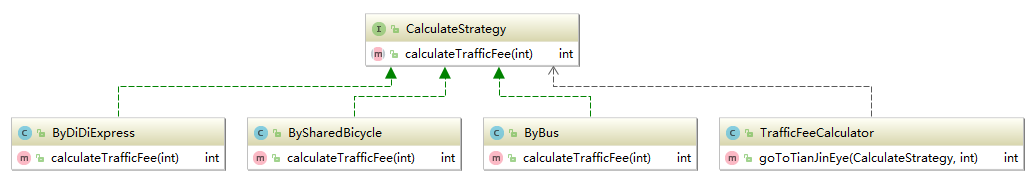# 如何实现

## 业务场景

2017年的2月14号，王二狗和牛翠花约好在天津之眼约定终身，二狗打扮一番后准备出发，此时问题来了：是坐公交去呢，还是打滴滴快车呢？天气看起来也不错，要不骑共享单车吧，省钱还锻炼身体。

## 普通实现

public class TrafficFeeCalculator {
public int goToTianJinEye(String way, int distance) {
int trafficFee = 0;
switch (way) {
case "bus":
if (distance < 10)
trafficFee = 4;
else
trafficFee = 6;
break;
case "didi":
if(distance<3)
trafficFee = 8;
else
trafficFee=8+(distance-3)*3;
break;
case "sharedBicyle":
trafficFee = 2;
break;
default:
break;
}
return trafficFee;
}
}

## 使用策略模式public interface CalculateStrategy {
int calculateTrafficFee(int distance);
}

//乘坐公交车算法
public class ByBus implements CalculateStrategy {
@Override
public int calculateTrafficFee(int distance) {
return distance<10?4:6;
}
}
//乘坐滴滴快车算法
public class ByDiDiExpress implements CalculateStrategy {
@Override
public int calculateTrafficFee(int distance) {
return distance<3?8:(8+(distance-3)*3);
}
}
//骑共享单车算法
public class BySharedBicycle implements CalculateStrategy {
@Override
public int calculateTrafficFee(int distance) {
return 2;
}
}

public class TrafficFeeCalculator {
...

public int goToTianJinEye(CalculateStrategy strategy,int distance){
return strategy.calculateTrafficFee(distance);
}
}

    public static void main(String[] args) {
TrafficFeeCalculator calculator=new TrafficFeeCalculator();
System.out.println(String.format("乘坐公交车到天津之眼的花费为：%d块人民币",
calculator.goToTianJinEye(new ByBus(),10)));
System.out.println(String.format("乘坐滴滴快车到天津之眼的花费为：%d块人民币",
calculator.goToTianJinEye(new ByDiDiExpress(),10)));
System.out.println(String.format("骑共享单车到天津之眼的花费为：%d块人民币",
calculator.goToTianJinEye(new BySharedBicycle(),10)));
}

乘坐公交车到天津之眼的花费为：6块人民币